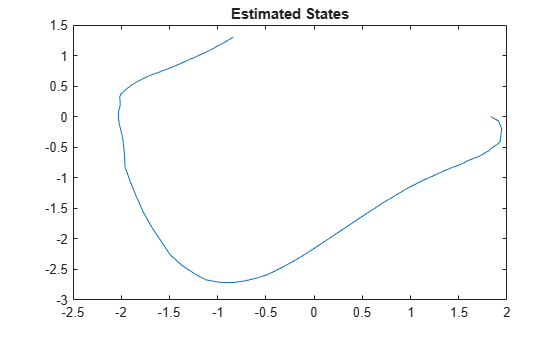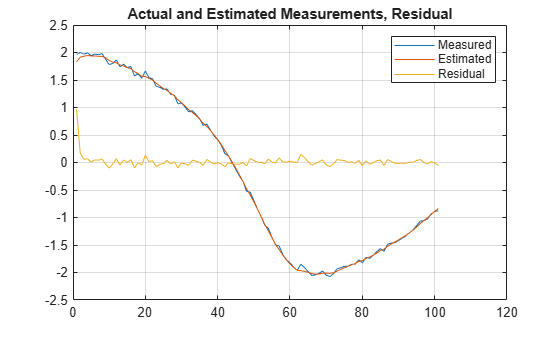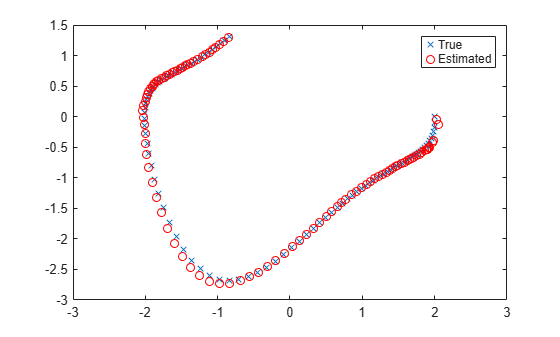# correct

Correct state and state estimation error covariance using extended or unscented Kalman filter, or particle filter and measurements

## Syntax

``````[CorrectedState,CorrectedStateCovariance] = correct(obj,y)``````
``````[CorrectedState,CorrectedStateCovariance] = correct(obj,y,Um1,...,Umn)``````

## Description

The `correct` command updates the state and state estimation error covariance of an `extendedKalmanFilter`, `unscentedKalmanFilter` or `particleFilter` object using measured system outputs. To implement extended or unscented Kalman filter, or particle filter, use the `correct` and `predict` commands together. If the current output measurement exists, you can use `correct` and `predict`. If the measurement is missing, you can only use `predict`. For information about the order in which to use the commands, see Using predict and correct Commands.

example

``````[CorrectedState,CorrectedStateCovariance] = correct(obj,y)``` corrects the state estimate and state estimation error covariance of an extended or unscented Kalman filter, or particle filter object `obj` using the measured output `y`.You create `obj` using the `extendedKalmanFilter`, `unscentedKalmanFilter` or `particleFilter` commands. You specify the state transition function and measurement function of your nonlinear system in `obj`. You also specify whether the process and measurement noise terms are additive or nonadditive in these functions. The `State` property of the object stores the latest estimated state value. Assume that at time step `k`, `obj.State` is $\stackrel{^}{x}\left[k|k-1\right]$. This value is the state estimate for time `k`, estimated using measured outputs until time `k-1`. When you use the `correct` command with measured system output `y[k]`, the software returns the corrected state estimate $\stackrel{^}{x}\left[k|k\right]$ in the `CorrectedState` output. Where $\stackrel{^}{x}\left[k|k\right]$ is the state estimate at time `k`, estimated using measured outputs until time `k`. The command returns the state estimation error covariance of $\stackrel{^}{x}\left[k|k\right]$ in the `CorrectedStateCovariance` output. The software also updates the `State` and `StateCovariance` properties of `obj` with these corrected values.Use this syntax if the measurement function h that you specified in `obj.MeasurementFcn` has one of the following forms: `y(k) = h(x(k))` — for additive measurement noise.`y(k) = h(x(k),v(k))` — for nonadditive measurement noise. Where `y(k)`, `x(k)`, and `v(k)` are the measured output, states, and measurement noise of the system at time step `k`. The only inputs to h are the states and measurement noise.```

example

``````[CorrectedState,CorrectedStateCovariance] = correct(obj,y,Um1,...,Umn)``` specifies additional input arguments, if the measurement function of the system requires these inputs. You can specify multiple arguments.Use this syntax if the measurement function h has one of the following forms: `y(k) = h(x(k),Um1,...,Umn)` — for additive measurement noise.`y(k) = h(x(k),v(k),Um1,...,Umn)` — for nonadditive measurement noise. `correct` command passes these inputs to the measurement function to calculate the estimated outputs.```

## Examples

collapse all

Estimate the states of a van der Pol oscillator using an extended Kalman filter algorithm and measured output data. The oscillator has two states and one output.

Create an extended Kalman filter object for the oscillator. Use previously written and saved state transition and measurement functions, `vdpStateFcn.m` and `vdpMeasurementFcn.m`. These functions describe a discrete-approximation to a van der Pol oscillator with the nonlinearity parameter $\mathrm{mu}$ equal to 1. The functions assume additive process and measurement noise in the system. Specify the initial state values for the two states as [1;0]. This is the guess for the state value at initial time `k`, based on knowledge of system outputs until time `k-1`, $\underset{}{\overset{ˆ}{x}}\left[k|k-1\right]$.

`obj = extendedKalmanFilter(@vdpStateFcn,@vdpMeasurementFcn,[1;0]);`

Load the measured output data `y` from the oscillator. In this example, use simulated static data for illustration. The data is stored in the `vdp_data.mat` file.

`load vdp_data.mat y`

Specify the process noise and measurement noise covariances of the oscillator.

```obj.ProcessNoise = 0.01; obj.MeasurementNoise = 0.16;```

Initialize arrays to capture results of the estimation.

```residBuf = []; xcorBuf = []; xpredBuf = [];```

Implement the extended Kalman filter algorithm to estimate the states of the oscillator by using the `correct` and `predict` commands. You first correct $\underset{}{\overset{ˆ}{x}}\left[k|k-1\right]$ using measurements at time `k` to get $\underset{}{\overset{ˆ}{x}}\left[k|k\right]$. Then, you predict the state value at the next time step $\underset{}{\overset{ˆ}{x}}\left[k+1|k\right]$ using $\underset{}{\overset{ˆ}{x}}\left[k|k\right]$, the state estimate at time step `k` that is estimated using measurements until time `k`.

To simulate real-time data measurements, use the measured data one time step at a time. Compute the residual between the predicted and actual measurement to assess how well the filter is performing and converging. Computing the residual is an optional step. When you use `residual`, place the command immediately before the `correct` command. If the prediction matches the measurement, the residual is zero.

After you perform the real-time commands for the time step, buffer the results so that you can plot them after the run is complete.

```for k = 1:size(y) [Residual,ResidualCovariance] = residual(obj,y(k)); [CorrectedState,CorrectedStateCovariance] = correct(obj,y(k)); [PredictedState,PredictedStateCovariance] = predict(obj); residBuf(k,:) = Residual; xcorBuf(k,:) = CorrectedState'; xpredBuf(k,:) = PredictedState'; end```

When you use the `correct` command, `obj.State` and `obj.StateCovariance` are updated with the corrected state and state estimation error covariance values for time step `k`, `CorrectedState` and `CorrectedStateCovariance`. When you use the `predict` command, `obj.State` and `obj.StateCovariance` are updated with the predicted values for time step `k+1`, `PredictedState` and `PredictedStateCovariance`. When you use the `residual` command, you do not modify any `obj` properties.

In this example, you used `correct` before `predict` because the initial state value was $\underset{}{\overset{ˆ}{x}}\left[k|k-1\right]$, a guess for the state value at initial time `k` based on system outputs until time `k-1`. If your initial state value is $\underset{}{\overset{ˆ}{x}}\left[k-1|k-1\right]$, the value at previous time `k-1` based on measurements until `k-1`, then use the `predict` command first. For more information about the order of using `predict` and `correct`, see Using predict and correct Commands.

Plot the estimated states, using the postcorrection values.

```plot(xcorBuf(:,1), xcorBuf(:,2)) title('Estimated States')```Plot the actual measurement, the corrected estimated measurement, and the residual. For the measurement function in `vdpMeasurementFcn`, the measurement is the first state.

```M = [y,xcorBuf(:,1),residBuf]; plot(M) grid on title('Actual and Estimated Measurements, Residual') legend('Measured','Estimated','Residual')```The estimate tracks the measurement closely. After the initial transient, the residual remains relatively small throughout the run.

Load the van der Pol ODE data, and specify the sample time.

`vdpODEdata.mat` contains a simulation of the van der Pol ODE with nonlinearity parameter mu=1, using ode45, with initial conditions `[2;0]`. The true state was extracted with sample time `dt = 0.05`.

```addpath(fullfile(matlabroot,'examples','ident','main')) % add example data load ('vdpODEdata.mat','xTrue','dt') tSpan = 0:dt:5;```

Get the measurements. For this example, a sensor measures the first state with a Gaussian noise with standard deviation `0.04`.

```sqrtR = 0.04; yMeas = xTrue(:,1) + sqrtR*randn(numel(tSpan),1);```

Create a particle filter, and set the state transition and measurement likelihood functions.

`myPF = particleFilter(@vdpParticleFilterStateFcn,@vdpMeasurementLikelihoodFcn);`

Initialize the particle filter at state `[2; 0]` with unit covariance, and use `1000` particles.

`initialize(myPF,1000,[2;0],eye(2));`

Pick the `mean` state estimation and `systematic` resampling methods.

```myPF.StateEstimationMethod = 'mean'; myPF.ResamplingMethod = 'systematic';```

Estimate the states using the `correct` and `predict` commands, and store the estimated states.

```xEst = zeros(size(xTrue)); for k=1:size(xTrue,1) xEst(k,:) = correct(myPF,yMeas(k)); predict(myPF); end```

Plot the results, and compare the estimated and true states.

```figure(1) plot(xTrue(:,1),xTrue(:,2),'x',xEst(:,1),xEst(:,2),'ro') legend('True','Estimated')````rmpath(fullfile(matlabroot,'examples','ident','main')) % remove example data`

Consider a nonlinear system with input `u` whose state `x` and measurement `y` evolve according to the following state transition and measurement equations:

`$x\left[k\right]=\sqrt{x\left[k-1\right]+u\left[k-1\right]}+w\left[k-1\right]$`

`$y\left[k\right]=x\left[k\right]+2*u\left[k\right]+v\left[k{\right]}^{2}$`

The process noise `w` of the system is additive while the measurement noise `v` is nonadditive.

Create the state transition function and measurement function for the system. Specify the functions with an additional input `u`.

```f = @(x,u)(sqrt(x+u)); h = @(x,v,u)(x+2*u+v^2);```

`f` and `h` are function handles to the anonymous functions that store the state transition and measurement functions, respectively. In the measurement function, because the measurement noise is nonadditive, `v` is also specified as an input. Note that `v` is specified as an input before the additional input `u`.

Create an extended Kalman filter object for estimating the state of the nonlinear system using the specified functions. Specify the initial value of the state as 1 and the measurement noise as nonadditive.

`obj = extendedKalmanFilter(f,h,1,'HasAdditiveMeasurementNoise',false);`

Specify the measurement noise covariance.

`obj.MeasurementNoise = 0.01;`

You can now estimate the state of the system using the `predict` and `correct` commands. You pass the values of `u` to `predict` and `correct`, which in turn pass them to the state transition and measurement functions, respectively.

Correct the state estimate with measurement `y`[`k`]=`0.8` and input `u`[`k`]=`0.2` at time step `k`.

`correct(obj,0.8,0.2)`

Predict the state at the next time step, given `u`[`k`]=`0.2`.

`predict(obj,0.2)`

Retrieve the error, or residual, between the prediction and the measurement.

`[Residual, ResidualCovariance] = residual(obj,0.8,0.2);`

## Input Arguments

collapse all

Extended or unscented Kalman filter, or particle filter object for online state estimation, created using one of the following commands:

Measured system output at the current time step, specified as an N-element vector, where N is the number of measurements.

Additional input arguments to the measurement function of the system, specified as input arguments of any type. The measurement function, h, is specified in the `MeasurementFcn` or `MeasurementLikelihoodFcn` property of `obj`. If the function requires input arguments in addition to the state and measurement noise values, you specify these inputs in the `correct` command syntax. `correct` command passes these inputs to the measurement or the measurement likelihood function to calculate estimated outputs. You can specify multiple arguments.

For example, suppose that your measurement or measurement likelihood function calculates the estimated system output `y` using system inputs `u` and current time `k`, in addition to the state `x`:

`y(k) = h(x(k),u(k),k)`

Then when you perform online state estimation at time step `k`, specify these additional inputs in the `correct` command syntax:

`[CorrectedState,CorrectedStateCovariance] = correct(obj,y,u(k),k);`

## Output Arguments

collapse all

Corrected state estimate, returned as a vector of size M, where M is the number of states of the system. If you specify the initial states of `obj` as a column vector then M is returned as a column vector, otherwise M is returned as a row vector.

For information about how to specify the initial states of the object, see the `extendedKalmanFilter`, `unscentedKalmanFilter` and `particleFilter` reference pages.

Corrected state estimation error covariance, returned as an M-by-M matrix, where M is the number of states of the system.

collapse all

### Using `predict` and `correct` Commands

After you have created an extended or unscented Kalman filter, or particle filter object, `obj`, to implement the estimation algorithms, use the `correct` and `predict` commands together.

At time step `k`, `correct` command returns the corrected value of states and state estimation error covariance using measured system outputs `y[k]` at the same time step. If your measurement function has additional input arguments `Um`, you specify these as inputs to the `correct` command. The command passes these values to the measurement function.

`[CorrectedState,CorrectedCovariance] = correct(obj,y,Um)`

The `correct` command updates the `State` and `StateCovariance` properties of the object with the estimated values, `CorrectedState` and `CorrectedCovariance`.

The `predict` command returns the prediction of state and state estimation error covariance at the next time step. If your state transition function has additional input arguments `Us`, you specify these as inputs to the `predict` command. The command passes these values to the state transition function.

`[PredictedState,PredictedCovariance] = predict(obj,Us)`

The `predict` command updates the `State` and `StateCovariance` properties of the object with the predicted values, `PredictedState` and `PredictedCovariance`.

If the current output measurement exists at a given time step, you can use `correct` and `predict`. If the measurement is missing, you can only use `predict`. For details about how these commands implement the algorithms, see Extended and Unscented Kalman Filter Algorithms for Online State Estimation.

The order in which you implement the commands depends on the availability of measured data `y`, `Us`, and `Um` for your system:

• `correct` then `predict` — Assume that at time step `k`, the value of `obj.State` is $\stackrel{^}{x}\left[k|k-1\right]$. This value is the state of the system at time `k`, estimated using measured outputs until time `k-1`. You also have the measured output `y[k]` and inputs `Us[k]` and `Um[k]` at the same time step.

Then you first execute the `correct` command with measured system data `y[k]` and additional inputs `Um[k]`. The command updates the value of `obj.State` to be $\stackrel{^}{x}\left[k|k\right]$, the state estimate for time `k`, estimated using measured outputs up to time `k`. When you then execute the `predict` command with input `Us[k]`, `obj.State` now stores $\stackrel{^}{x}\left[k+1|k\right]$. The algorithm uses this state value as an input to the `correct` command in the next time step.

• `predict` then `correct` — Assume that at time step `k`, the value of `obj.State` is $\stackrel{^}{x}\left[k-1|k-1\right]$. You also have the measured output `y[k]` and input `Um[k]` at the same time step but you have `Us[k-1]` from the previous time step.

Then you first execute the `predict` command with input `Us[k-1]`. The command updates the value of `obj.State` to $\stackrel{^}{x}\left[k|k-1\right]$. When you then execute the `correct` command with input arguments `y[k]` and `Um[k]`, `obj.State` is updated with $\stackrel{^}{x}\left[k|k\right]$. The algorithm uses this state value as an input to the `predict` command in the next time step.

Thus, while in both cases the state estimate for time `k`, $\stackrel{^}{x}\left[k|k\right]$ is the same, if at time `k` you do not have access to the current state transition function inputs `Us[k]`, and instead have `Us[k-1]`, then use `predict` first and then `correct`.

For an example of estimating states using the `predict` and `correct` commands, see Estimate States Online Using Extended Kalman Filter or Estimate States Online using Particle Filter.

#### Introduced in R2016b

##### SupportGet trial now